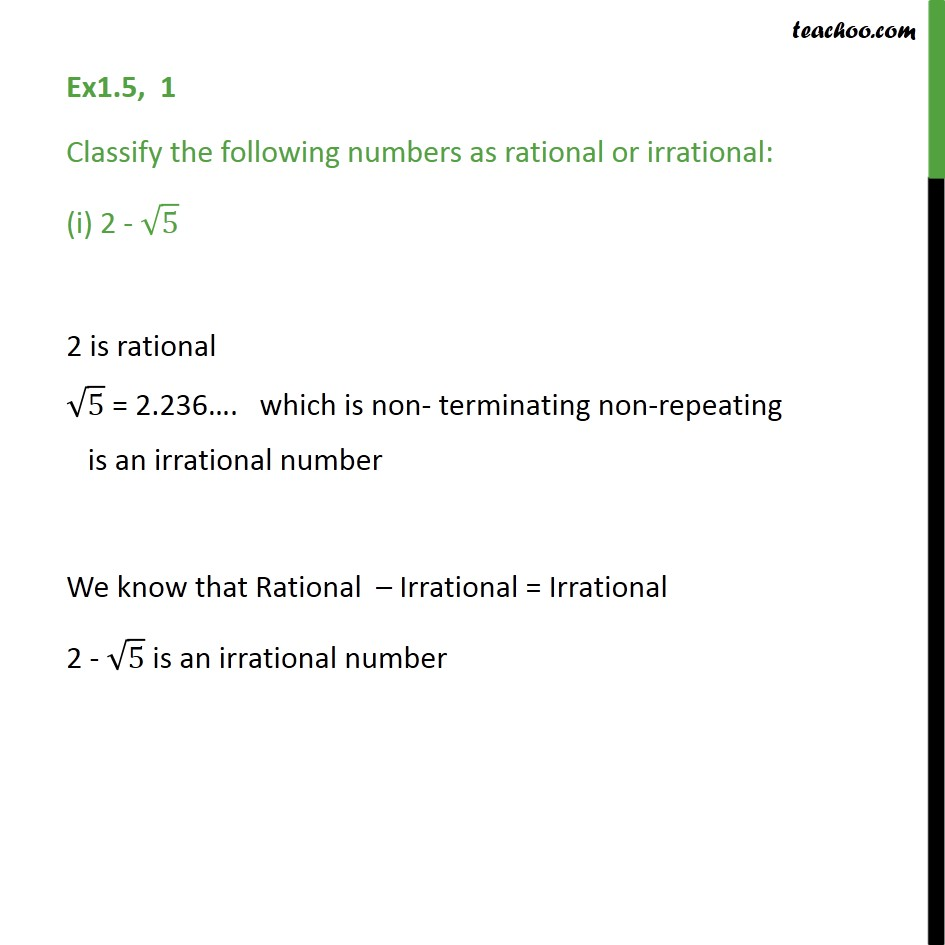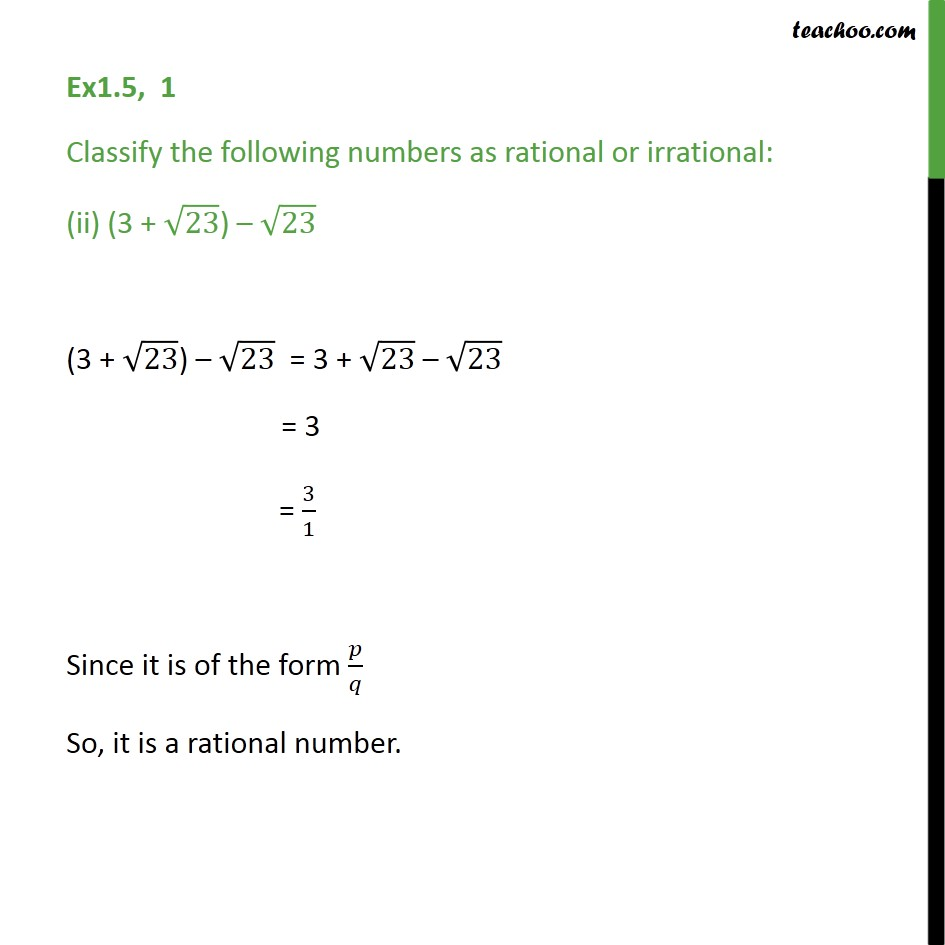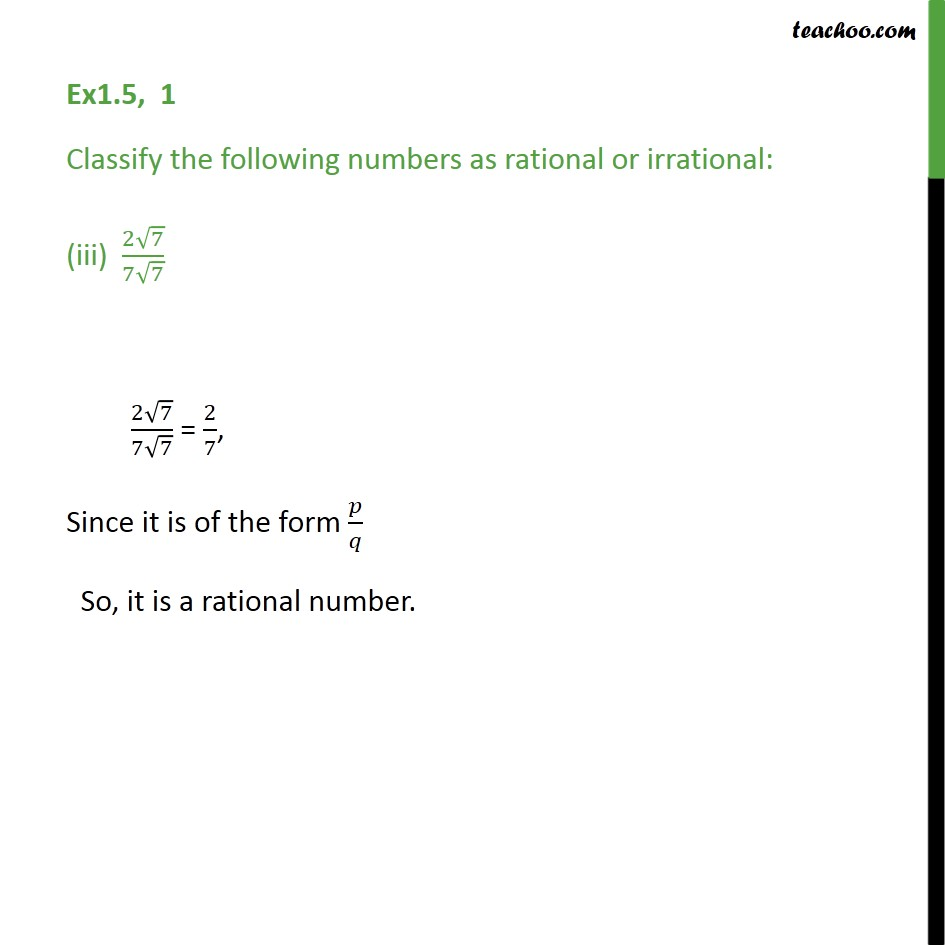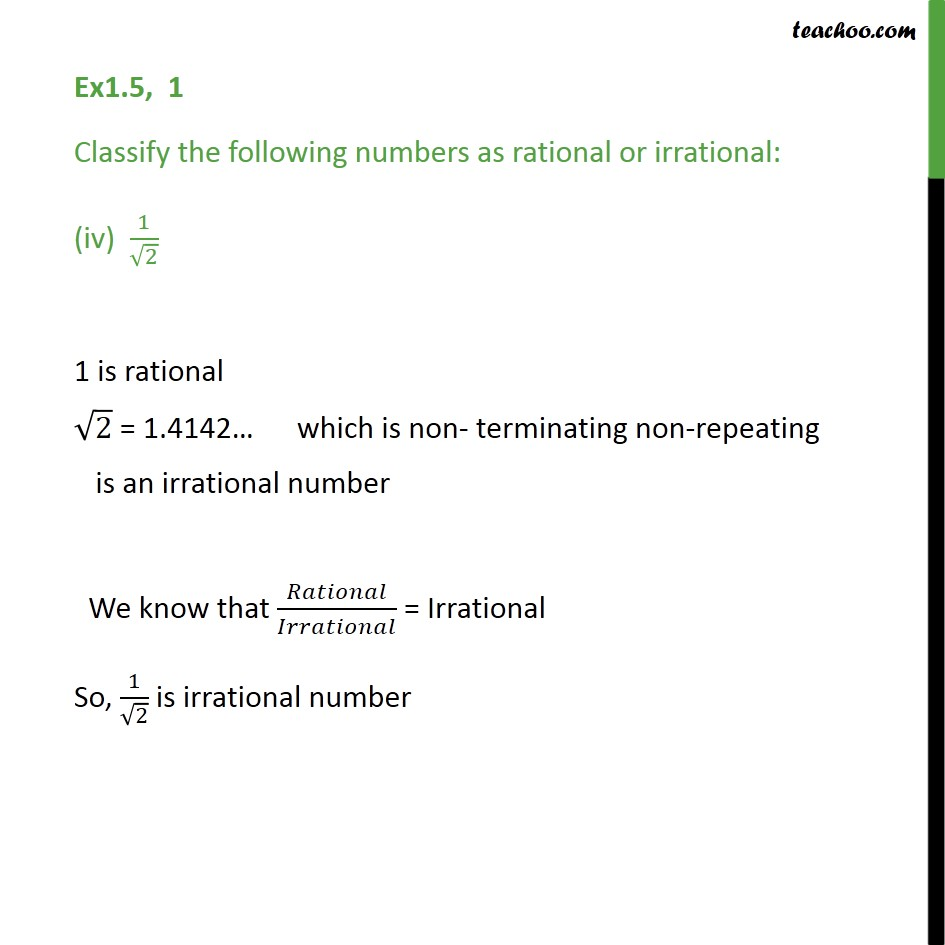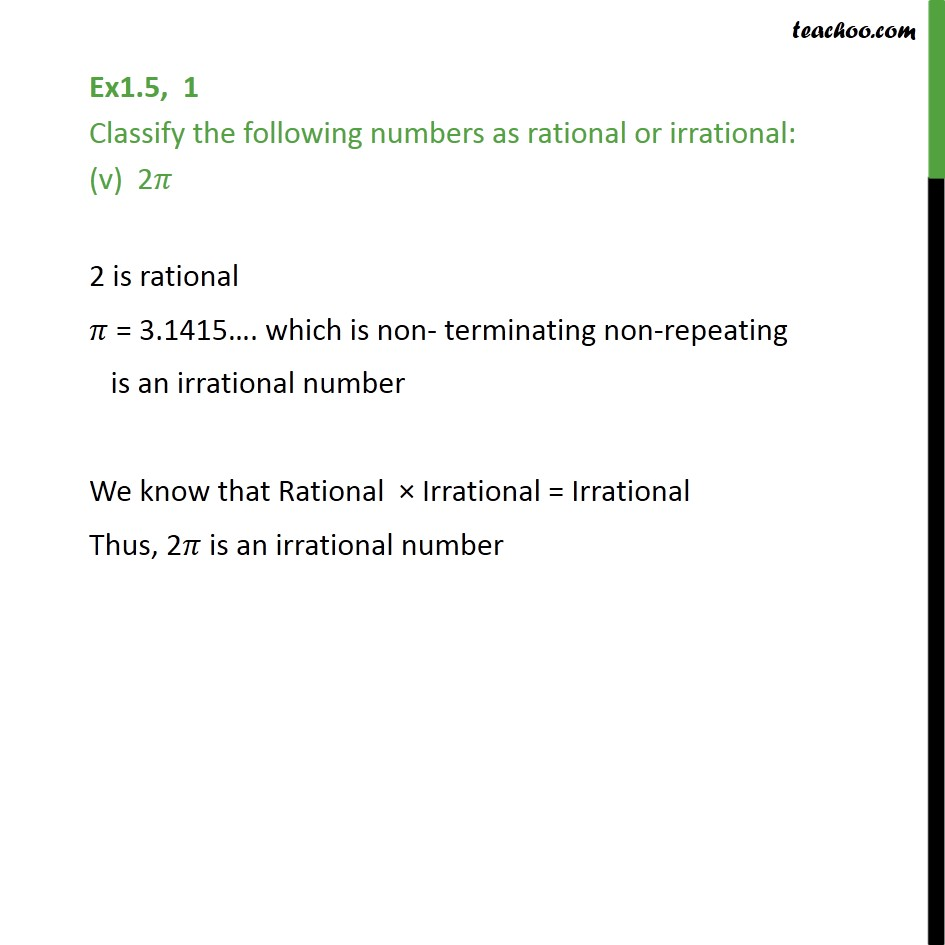1. Chapter 1 Class 9 Number Systems
2. Serial order wise
3. Ex 1.5

Transcript

Ex1.5, 1 Classify the following numbers as rational or irrational: (i) 2 - 5 2 is rational 5 = 2.236 . which is non- terminating non-repeating is an irrational number We know that Rational Irrational = Irrational 2 - 5 is an irrational number Ex1.5, 1 Classify the following numbers as rational or irrational: (ii) (3 + 23) 23 (3 + 23) 23 = 3 + 23 23 = 3 = 3/1 Since it is of the form / So, it is a rational number. Ex1.5, 1 Classify the following numbers as rational or irrational: (iii) (2 7)/(7 7) (2 7)/(7 7) = 2/7, Since it is of the form / So, it is a rational number. Ex1.5, 1 Classify the following numbers as rational or irrational: (iv) 1/ 2 1 is rational 2 " = 1.4142 " which is non- terminating non-repeating is an irrational number We know that / = Irrational So, 1/ 2 is irrational number Ex1.5, 1 Classify the following numbers as rational or irrational: (v) 2 2 is rational = 3.1415 . which is non- terminating non-repeating is an irrational number We know that Rational Irrational = Irrational Thus, 2 is an irrational number

Ex 1.5